## RD Sharma Class 8 Solutions Chapter 3 Squares and Square Roots Ex 3.8

These Solutions are part of RD Sharma Class 8 Solutions. Here we have given RD Sharma Class 8 Solutions Chapter 3 Squares and Square Roots Ex 3.8

Other Exercises

Question 1.
Find the square root of each of the following correct to three places of decimal :
(i) 5
(ii) 7
(iii) 17
(iv) 20
(v) 66
(vi) 427
(vii) 1.7
(vii’) 23.1
(ix) 2.5
(x) 237.615
(xi) 15.3215
(xii) 0.9
(xiii) 0.1
(xiv) 0.016
(xv) 0.00064
(xvi) 0.019
(xvii) $$\frac {7 }{ 8 }$$
(xviii) $$\frac {5 }{ 12 }$$
(xix) 2 $$\frac {1 }{ 2 }$$
(xx) 287 $$\frac {8 }{ 8 }$$
Solution: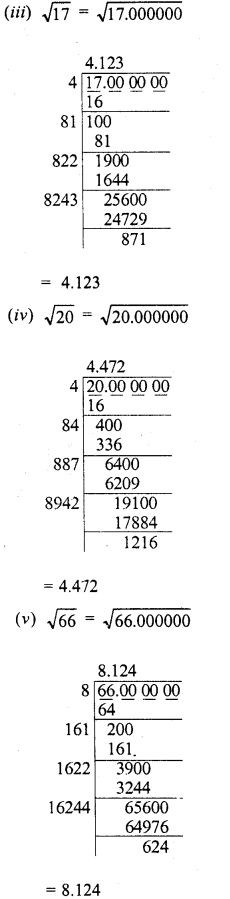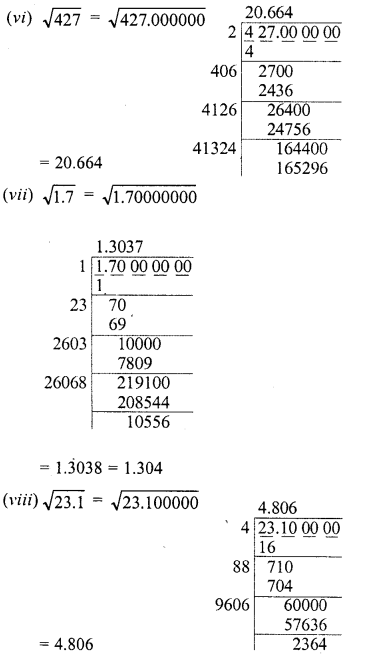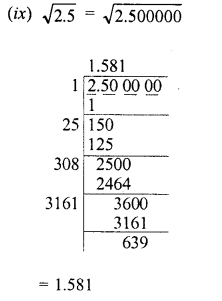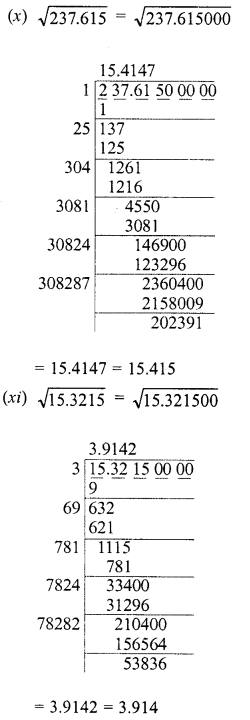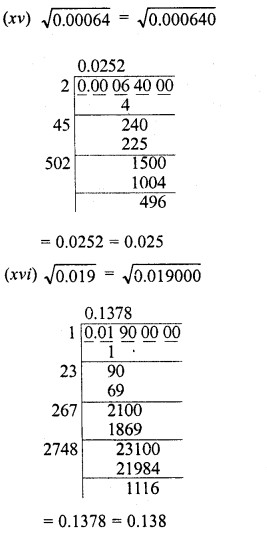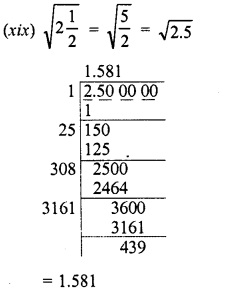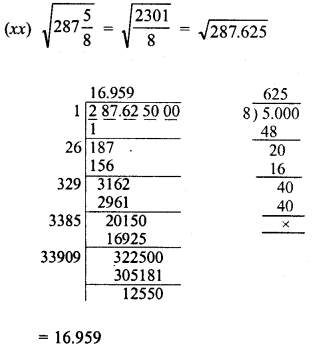Question 2.
Find the square root of 12.0068 correct to four decimal places.
Solution:Question 3.
Find the square root of 11 correct to five decimal places.
Solution: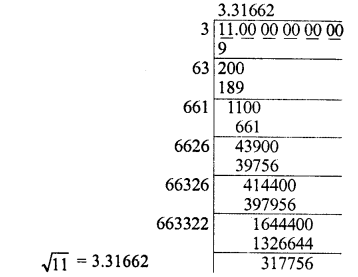Question 4.
Given that √2, = 1-414, √3 = 1.732, √5 = 2.236 and √7 = 2.646. Evaluate each of the following :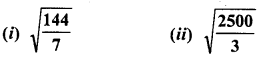Solution: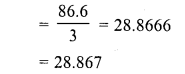Question 5.
Given that √2 = 1-414, √3 = 1-732, √5= 2.236 and √7= 2.646, find the square roots of the following :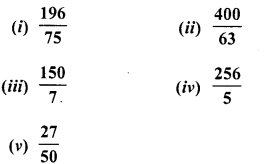Solution: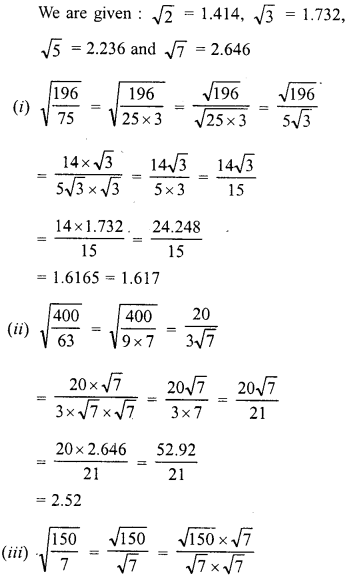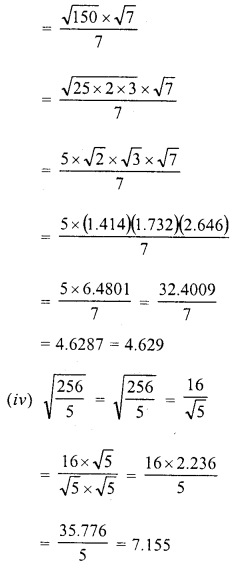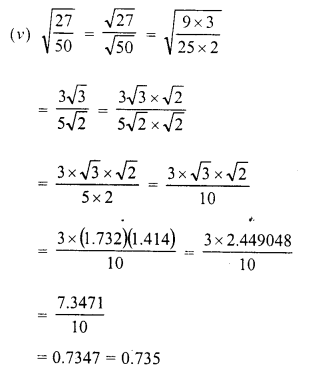Hope given RD Sharma Class 8 Solutions Chapter 3 Squares and Square Roots Ex 3.8 are helpful to complete your math homework.

If you have any doubts, please comment below. Learn Insta try to provide online math tutoring for you.